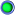View Single Post06-17-2019, 12:16 AM   #489
Fabian
Human being with feelings

Join Date: Sep 2008
Location: Sweden
Posts: 5,517Quote:
 Originally Posted by daneriusHi. Im having trouble understanding this bit of code. Its from Losers Waveshaping Distortion. Mostly the second line "hdistr =....". How does the "100,.999" bit work? I get that It gives a value for "foo" to be calculated and then used in the formula. How would I enter these values in a regular calculator? Slider range is 0-100. /thx @slider hdistr = min(slider1/100,.999); foo = 2*hdistr/(1-hdistr); @sample spl0 = min(max(spl0,-1),1); spl1 = min(max(spl1,-1),1); spl0 = (1+foo)*spl0/(1+foo*abs(spl0)); spl1 = (1+foo)*spl1/(1+foo*abs(spl1));
I am not sure exactly what you are asking. Do you mean
Code:
`min(slider1/100,.999)`
It divides the value of slider1 by 100, then takes the minimum of that value and 0.999. Is this what you mean?

Or do you mean to ask why the value of foo is calculated the way it is? Of that, I have no idea, sorry.
__________________
// MVHMF
I never always did the right thing, but all I did wasn't wrong...